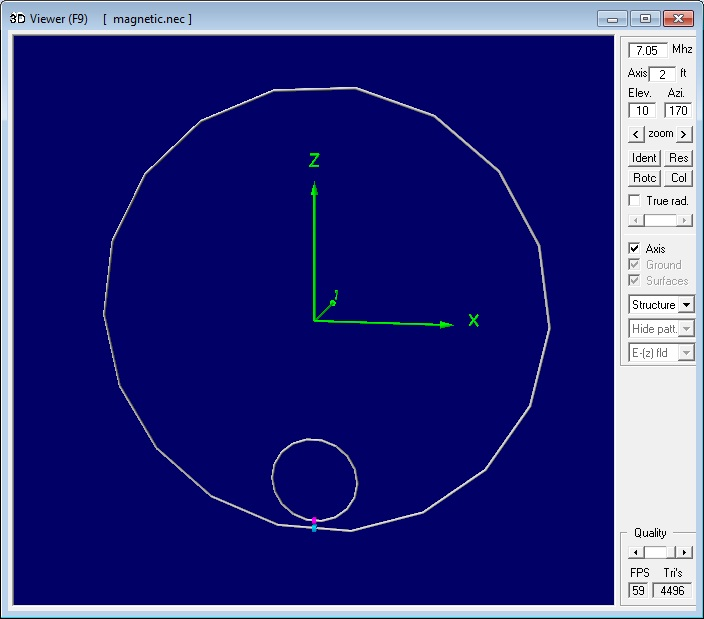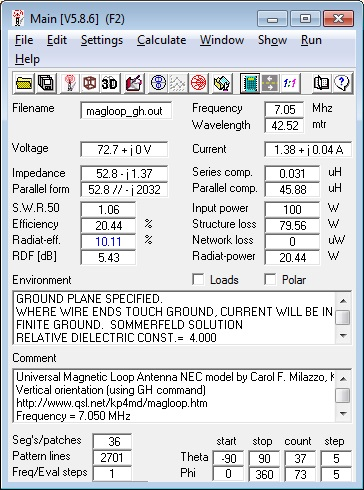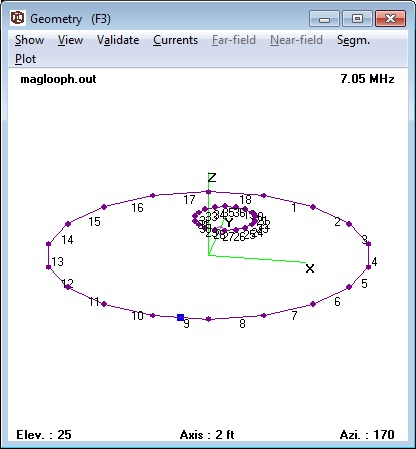# A Universal HF Magnetic Loop Antenna NEC Model

## Design of a NEC Model for Small High Frequency Magnetic Loop Antennas

by Dr. Carol F. Milazzo, KP4MD (posted 16 September 2011)
E-mail: [email protected]

### SUMMARY

This article describes an application of NEC modeling to design and analyze the performance of high frequency magnetic loop antennas with loop coupling.

### INTRODUCTION

The small magnetic loop has received growing interest as a relatively efficient compact high frequency antenna for limited spaces.  These antennas feature a radiating conductor in a loop of either circular or polygon shape of a circumference between 0.1λ and 0.25λ resonated to the operating frequency with a capacitor.  These notes describe a NEC model designed for analyzing and optimizing a loop-coupled small magnetic loop antenna in an equilateral polygonal configuration.

### VERTICAL MAGNETIC LOOP ANTENNA MODEL

The 4nec2 modeling program1 contains a model of an 18-sided vertical magnetic loop with an 18-sided coupling loop in the sample file named "models\HFshort\Magnetic.nec".  This is a free space model with fixed coordinates and perfect conductors.  When run with 4nec2, this model is resonant at 7.05 MHz with an impedance of 1.15 ohms and a radiation efficiency of 100%.  With copper wire conductivity specified, the model yields clearly erroneous results, with negative calculated efficiency and structure loss that exceeds the input power.  This model fails the average gain validation test2 with an AGT result of 4.86.

I decided to use NEC variables and functions to design a new model that would simulate an equilateral polygonal magnetic loop antenna of variable dimensions and at varying heights over a simulated ground.  This would facilitate the use of the optimizer function to maximize impedance matching and efficiency in real world situations.

The radius (distance from center to vertices) and number of sides (from 3 to 18) of the loops are specified and then the coordinates of the segment are calculated using the sine and cosine values for each angle increment about the center of each loop.Figure 1. The 4nec2 sample "Magnetic.nec" model
fails validation with the Average Gain Test.

CONTENTS

Table 1.  NEC Model Variable Definitions
Variable definitions
Main Loop
Coupling Loop
Loop diameter (input feet)
d
d2
r
r2
Loop wire braid diameter (input)
dia
dia2
Loop wire outer diameter (input) od
od2
Number of polygon sides (input)
n
n2
Angle increment (calculated as 360°/n)
inc
inc2
Segments per wire
seg
seg2
Height of loop center above ground
ht
ht2
Height of main loop base above ground
h

Distance between tops of loops

h2
Operating frequency = frq
frq

Resonating capacitance = C
c

Capacitor Q=2000 (typical) qc

Capacitor resistance Rc (calculated) rc

Figure 2 shows the model of a 6.5 foot diameter vertical magnetic loop with an operating frequency of 7.05 MHz.  The main loop is RG-8A/U coaxial cable (outer diameter 0.405 inches) and the coupling loop is RG-58/U (outer diameter 0.195 inches).  Wires 1 through 18 comprise the main loop and wires 19 through 38 comprise the coupling loop.  The resonating capacitor is on wire 9 and the feed point is on wire 27.  Optimizing for 50 ohm impedance and maximum radiation efficiency (10.1%) yielded the loop dimensions in feet listed in Table 2.  Changing the main loop from RG-8A/U cable (conductivity 4,500,000 mhos/m) to a solid copper tube of the same diameter (conductivity 58,000,000 mhos/m) would increase the radiation efficiency to 36.5%.  This model passes the average gain validation test with an AGT result of 1.0 (-0 dB).  It was noted that the coupling loop diameter, wire radius and loop separation distance all influenced the calculated input impedance.  For any specified coupling loop wire radius, only one specific combination of coupling loop diameter and separation from the main loop yielded the non-reactive 50 ohm impedance match.  Download the Vertical Magnetic Loop Antenna NEC file.

Table 2.  Magnetic Loop Antenna NEC Model Calculations
*Values for Horizontal Loop in Parentheses
Variable values
Main Loop
Coupling Loop
Loop diameter (input feet)
d=6.5
d2=1.84 (1.61)*
r=3.25
r2=0.92 (0.80)*
Loop wire braid diameter (input inches)
dia=0.32
dia2=0.135
Loop wire braid radius (calculated feet)
Loop wire outer diameter (input inches) od=0.405
od2=0.195
Number of polygon sides (input)
n=18
n2=18
Angle increment (calculated as 360°/n)
inc=20
inc2=20
Segments per wire seg=1
seg2=1
Height of loop center above ground (feet)
ht=15.25 (12)*
ht2=17.40 (12)*
Height of main loop base above ground (feet)
h=12

Distance between tops of loops (feet)

h2=0.19 (0.14)*
Operating frequency frq=7.05 MHz
frq

Resonating capacitance pF
c=53.7 (53.6)*

Capacitor Q=2000 (typical)
qc

Capacitor resistance Rc (calculated) rc

When designing loops with fewer than 18 sides, the corresponding unused numbered wires would be deleted from this NEC model.  During design, the voltage source is first placed on the wire of the main loop opposite from the capacitor side and the capacitor is optimized for zero reactance to resonate the loop on the operating frequency.  The voltage source is then moved to appropriate wire on the coupling loop.

### HORIZONTAL MAGNETIC LOOP ANTENNA MODEL

Figure 4 lists the NEC calculations for the loop in the horizontal orientation.  At 12 feet above the ground, the coupling loop needed to be resized to 1.61 feet diameter and 0.14 feet separation to restore the non-reactive 50 ohm impedance and the radiation efficiency was a mere 1.7%.  The radiation efficiency increased to a maximum 9.5% by raising the antenna to a height of 59 feet with the optimizer function.  Download the Horizontal Magnetic Loop Antenna NEC file.

### CAUTIONS

The following NEC-2 modeling limitations should be considered when interpreting these models:

1. If the circumference of the coupling loop is less than 0.07λ, the calculations may be questionable as NEC-2 is unable to model such small loops.3
2. The NEC-2 documentation specifically recommends that closely space parallel wires be arranged so that the segments are carefully matched.  In models where both the coupling and main loops are in close proximity, the disparities in the segment lengths of the loops may be significant.  This situation might be addressed by designing the coupling loop with fewer and longer sides.4
3. NEC-2 models of parallel wires of different diameters (as has been well established) produce highly erroneous values.  As the model predicts that main loop and coupling loop will be of different wire diameters to achieve impedance transformation, the significance of this guideline for parallel wires where portions of the loops are nearly parallel is uncertain.5
4. Loops with fewer than 8 sides will require increased segmentation of the wires in order to pass validation with the Average Gain Test.
5. Small changes to the number of sides of the coupling loop have little effect on the results; however, changes to the number of sides of the main loop will require readjustment of the capacitor to restore resonance prior to calculating performance due to perimeter variation.
6. Finally, I have validated models for the main loop against a physical antenna8.  Validation with the feed through the modeled coupling loop is pending.

### ZERO PITCH HELIX MODELS

Owen Duffy, VK1OD, suggested using the NEC GH command rather than the GW command to construct a loop as a helix with zero pitch.  In some NEC-2 programs as 4nec2, the GH command gives a divide by zero error when the helix spacing and length are set to zero; however, I found that the GH command will work in 4nec2 when these parameters are set to a very small value such as 1e-100.

The following NEC files that use the GH command produce models that yield identical performance calculations to the previous models.  Specification of the variables n and n2 can produce loops of any number of sides without requiring the addition or deletion of lines to the NEC file; however, each side will be comprised of only one segment.  Such loops with fewer than 8 sides fail validation with the Average Gain Test.

### ALTERNATIVE COUPLING METHODS

At times, the 4nec2 optimizer function does not converge on a best match of the 50 ohm transmission line to the magnetic loop through a simple coupling loop.  Various alternative coupling methods such as the Faraday loop, gamma match, and ferrite transformer all have their proponents with accompanying controversy.  Doerenberg, N4SPP, has compared and documented his experience with these various methods6 and recommends the ferrite toroid transformer for its simplicity while noting the need to vary the turn ratio in order to maintain an adequate impedance match over a 4:1 frequency range. Toroid core coupling also permits estimated measurement (through the transformation ratio) of the loop impedance. Goff and Milazzo have described the construction of magnetic loop antennas using toroid matching transformers with variable turn ratios.7,8

### REFERENCES

1. 4nec2 antenna modeling software, Voors, A.
2. "Modeling the Unmodelable", Cebik, LB, W4RNL, Antennex, July 2006
3. "Recent Advances to NEC: Applications and Validation", Burke, G, March 1989
4. "Dipoles: Variety and Modeling Hazards", Cebik, LB, W4RNL, Antennex, February 2007
6. Magnetic Loop Antenna for 80-40-30-20 mtr, Doerenberg, F, N4SPP
7. Portable Magnetic Loop Antenna Version Two, Goff, R, G4FON
8. 14-30 MHz Magnetic Loop Antenna, Milazzo, C, KP4MD
9. Small Transmitting Loop Antennas, Yates, S, AA5T

### APPENDIX: NEC Model FilesFigure 2. Vertical Magnetic Loop ModelFigure 3. Vertical Magnetic Loop CalculationsFigure 4. Horizontal Magnetic Loop ModelFigure 5. Horizontal Magnetic Loop Calculations